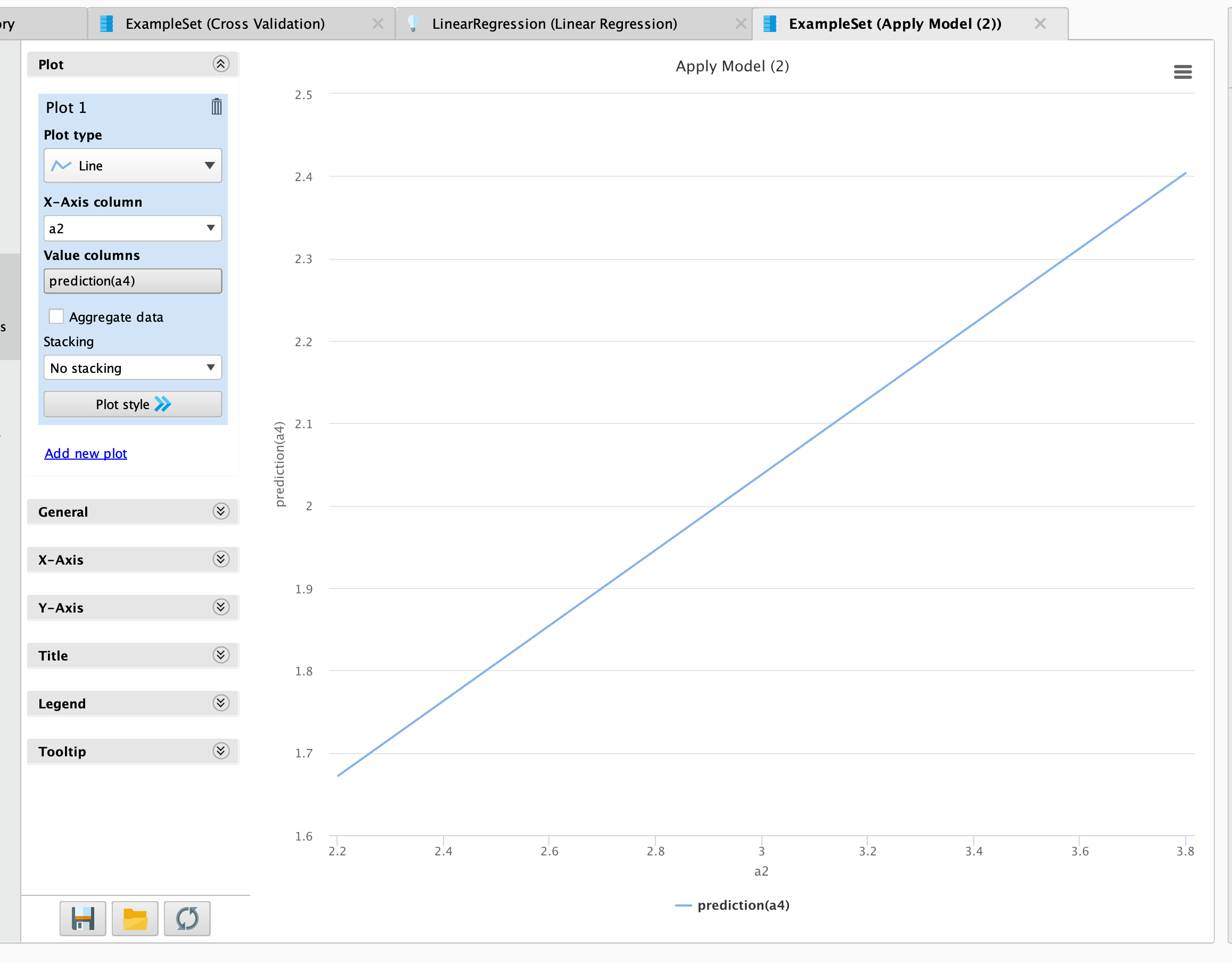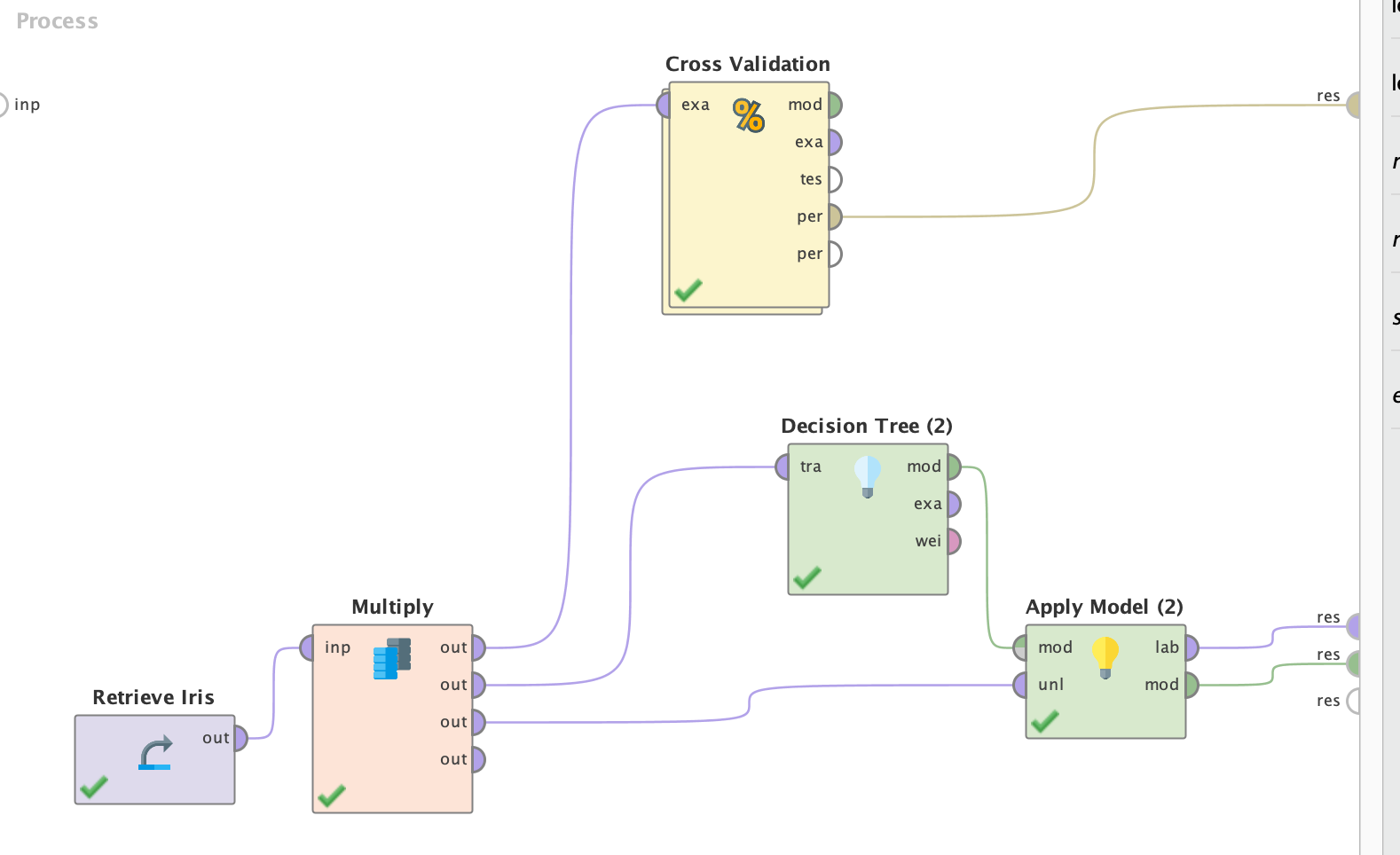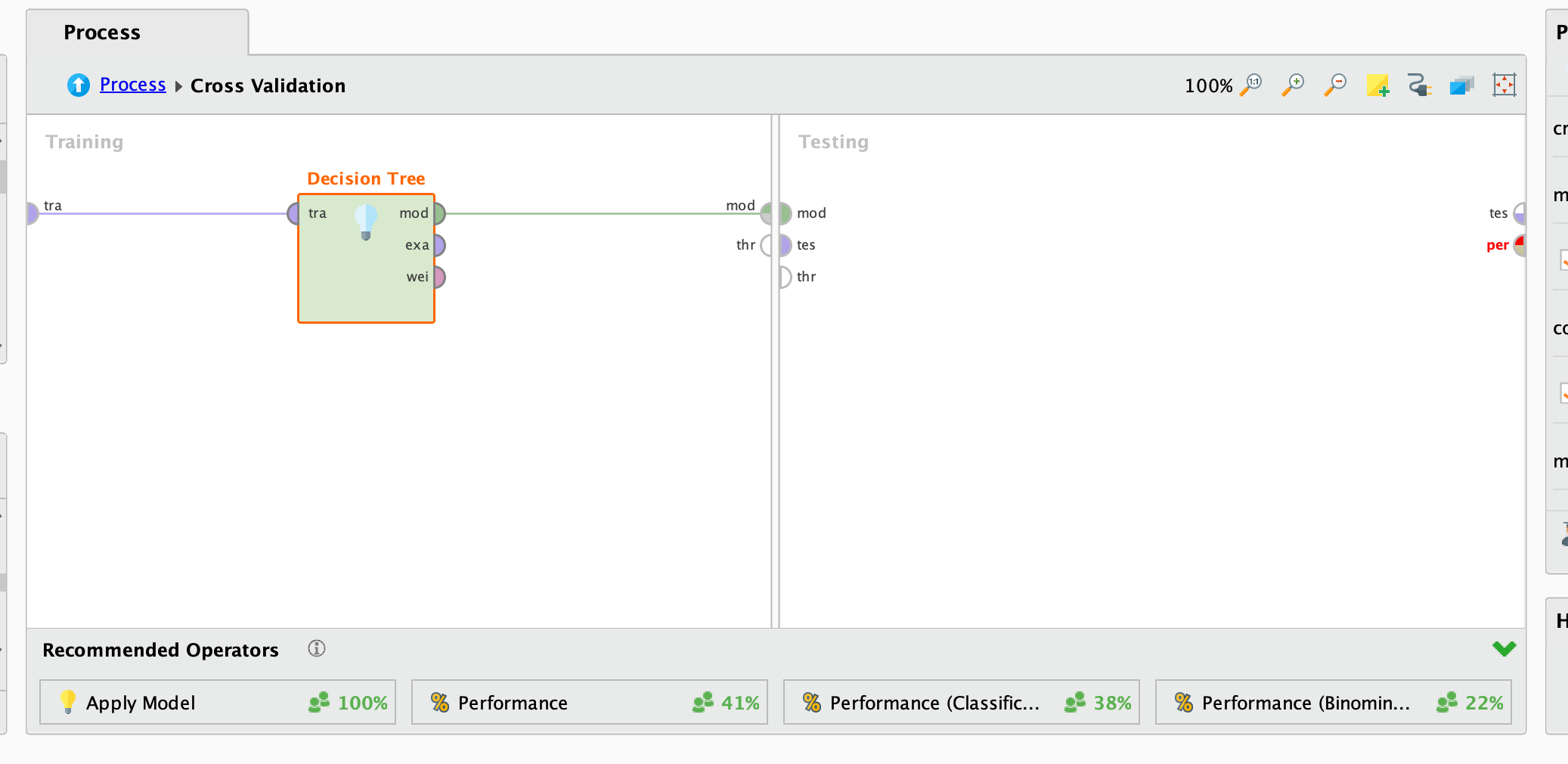# "Regression problem with cross-validation"

Member Posts: 9Contributor II
edited May 2019 in Help
Hi all

I have a concern about the output from cross-validation with regression.  The CV operator should break the data into (say) 10 segments and sequentially use each 10% of the data as a test set for a model built with the other 90% to measure performance - but when reporting out its model, that should be done with all the data, and the predictions made with the model using all the data.

That means that if you have a single attribute to use as a predictor, and plot the predicted value against this, you should get a straight line.

However, I get a jerky line.  This is specific to CV, if I try the same exercise with split validation it works fine.

Am I misunderstanding the way CV works or...?

To make it easier to see the problem I have adapted the Iris dataset to illustrate it, with this process:

<?xml version="1.0" encoding="UTF-8"?><process version="9.2.000">
<context>
<input/>
<output/>
<macros/>
</context>
<operator activated="true" class="process" compatibility="9.2.000" expanded="true" name="Process">
<parameter key="logverbosity" value="init"/>
<parameter key="random_seed" value="2001"/>
<parameter key="send_mail" value="never"/>
<parameter key="process_duration_for_mail" value="30"/>
<parameter key="encoding" value="SYSTEM"/>
<process expanded="true">
<operator activated="true" class="retrieve" compatibility="9.2.000" expanded="true" height="68" name="Retrieve Iris" width="90" x="45" y="85">
<parameter key="repository_entry" value="//Samples/data/Iris"/>
</operator>
<operator activated="true" class="filter_examples" compatibility="9.2.000" expanded="true" height="103" name="Filter Examples" width="90" x="179" y="85">
<parameter key="parameter_expression" value=""/>
<parameter key="condition_class" value="custom_filters"/>
<parameter key="invert_filter" value="false"/>
<list key="filters_list">
<parameter key="filters_entry_key" value="label.equals.Iris-virginica"/>
</list>
<parameter key="filters_logic_and" value="true"/>
</operator>
<operator activated="true" class="select_attributes" compatibility="9.2.000" expanded="true" height="82" name="Select Attributes" width="90" x="313" y="85">
<parameter key="attribute_filter_type" value="subset"/>
<parameter key="attribute" value="label"/>
<parameter key="attributes" value="a4|a2"/>
<parameter key="use_except_expression" value="false"/>
<parameter key="value_type" value="attribute_value"/>
<parameter key="use_value_type_exception" value="false"/>
<parameter key="except_value_type" value="time"/>
<parameter key="block_type" value="attribute_block"/>
<parameter key="use_block_type_exception" value="false"/>
<parameter key="except_block_type" value="value_matrix_row_start"/>
<parameter key="invert_selection" value="false"/>
<parameter key="include_special_attributes" value="true"/>
</operator>
<operator activated="true" class="set_role" compatibility="9.2.000" expanded="true" height="82" name="Set Role" width="90" x="447" y="85">
<parameter key="attribute_name" value="a4"/>
<parameter key="target_role" value="label"/>
</operator>
<operator activated="true" class="concurrency:cross_validation" compatibility="9.2.000" expanded="true" height="145" name="Cross Validation" width="90" x="380" y="238">
<parameter key="split_on_batch_attribute" value="false"/>
<parameter key="leave_one_out" value="false"/>
<parameter key="number_of_folds" value="10"/>
<parameter key="sampling_type" value="automatic"/>
<parameter key="use_local_random_seed" value="false"/>
<parameter key="local_random_seed" value="1992"/>
<parameter key="enable_parallel_execution" value="true"/>
<process expanded="true">
<operator activated="true" class="linear_regression" compatibility="9.2.000" expanded="true" height="103" name="Linear Regression" width="90" x="112" y="34">
<parameter key="feature_selection" value="M5 prime"/>
<parameter key="alpha" value="0.05"/>
<parameter key="max_iterations" value="10"/>
<parameter key="forward_alpha" value="0.05"/>
<parameter key="backward_alpha" value="0.05"/>
<parameter key="eliminate_colinear_features" value="true"/>
<parameter key="min_tolerance" value="0.05"/>
<parameter key="use_bias" value="true"/>
<parameter key="ridge" value="1.0E-8"/>
</operator>
<connect from_port="training set" to_op="Linear Regression" to_port="training set"/>
<connect from_op="Linear Regression" from_port="model" to_port="model"/>
<portSpacing port="source_training set" spacing="0"/>
<portSpacing port="sink_model" spacing="0"/>
<portSpacing port="sink_through 1" spacing="0"/>
</process>
<process expanded="true">
<operator activated="true" class="apply_model" compatibility="9.2.000" expanded="true" height="82" name="Apply Model" width="90" x="45" y="34">
<list key="application_parameters"/>
<parameter key="create_view" value="false"/>
</operator>
<operator activated="true" class="performance_regression" compatibility="9.2.000" expanded="true" height="82" name="Performance" width="90" x="179" y="34">
<parameter key="main_criterion" value="first"/>
<parameter key="root_mean_squared_error" value="false"/>
<parameter key="absolute_error" value="false"/>
<parameter key="relative_error" value="false"/>
<parameter key="relative_error_lenient" value="false"/>
<parameter key="relative_error_strict" value="false"/>
<parameter key="normalized_absolute_error" value="false"/>
<parameter key="root_relative_squared_error" value="false"/>
<parameter key="squared_error" value="false"/>
<parameter key="correlation" value="false"/>
<parameter key="squared_correlation" value="true"/>
<parameter key="prediction_average" value="false"/>
<parameter key="spearman_rho" value="false"/>
<parameter key="kendall_tau" value="false"/>
<parameter key="skip_undefined_labels" value="true"/>
<parameter key="use_example_weights" value="true"/>
</operator>
<connect from_port="model" to_op="Apply Model" to_port="model"/>
<connect from_port="test set" to_op="Apply Model" to_port="unlabelled data"/>
<connect from_op="Apply Model" from_port="labelled data" to_op="Performance" to_port="labelled data"/>
<connect from_op="Performance" from_port="performance" to_port="performance 1"/>
<connect from_op="Performance" from_port="example set" to_port="test set results"/>
<portSpacing port="source_model" spacing="0"/>
<portSpacing port="source_test set" spacing="0"/>
<portSpacing port="source_through 1" spacing="0"/>
<portSpacing port="sink_test set results" spacing="0"/>
<portSpacing port="sink_performance 1" spacing="0"/>
<portSpacing port="sink_performance 2" spacing="0"/>
</process>
</operator>
<operator activated="true" class="apply_model" compatibility="9.2.000" expanded="true" height="82" name="Apply Model (2)" width="90" x="581" y="238">
<list key="application_parameters"/>
<parameter key="create_view" value="false"/>
</operator>
<connect from_op="Retrieve Iris" from_port="output" to_op="Filter Examples" to_port="example set input"/>
<connect from_op="Filter Examples" from_port="example set output" to_op="Select Attributes" to_port="example set input"/>
<connect from_op="Select Attributes" from_port="example set output" to_op="Set Role" to_port="example set input"/>
<connect from_op="Set Role" from_port="example set output" to_op="Cross Validation" to_port="example set"/>
<connect from_op="Cross Validation" from_port="model" to_op="Apply Model (2)" to_port="model"/>
<connect from_op="Cross Validation" from_port="example set" to_op="Apply Model (2)" to_port="unlabelled data"/>
<connect from_op="Cross Validation" from_port="test result set" to_port="result 3"/>
<connect from_op="Cross Validation" from_port="performance 1" to_port="result 4"/>
<connect from_op="Apply Model (2)" from_port="labelled data" to_port="result 1"/>
<connect from_op="Apply Model (2)" from_port="model" to_port="result 2"/>
<portSpacing port="source_input 1" spacing="0"/>
<portSpacing port="sink_result 1" spacing="210"/>
<portSpacing port="sink_result 2" spacing="0"/>
<portSpacing port="sink_result 3" spacing="63"/>
<portSpacing port="sink_result 4" spacing="0"/>
<portSpacing port="sink_result 5" spacing="0"/>
</process>
</operator>
</process>

David
Tagged:

• 12Administrator, Moderator, Employee, RapidMiner Certified Analyst, Community Manager, Member, University Professor, PM Moderator Posts: 2,952Community Manager
Hi David -

Yes of course you should get a straight line plotting predicted(a4) vs a2, which I get when I run your process. Where do you see a jerky line?Scott
• Member Posts: 9Contributor II
Oops I forgot to mention something!  I added an additional Apply Model operator after Cross-validation to show what you should get, and that produces a straight line.  Now disable this second Apply Model and you will see the direct output from CV.  Many thanks Scott!
• Moderator, RapidMiner Certified Analyst, RapidMiner Certified Expert, Member Posts: 1,497Unicorn
Yes @sgenzer I think this would be a very helpful KB article. This question does come up a lot!
Brian T.
Lindon Ventures
Data Science Consulting from Certified RapidMiner Experts
• Member Posts: 9Contributor II
Many thanks Scott.  That's cracked it.  The workaround to insert a new Apply Model operator will work well and I will be able to explain to people why it is needed.  Very helpful!
DH
• 12Administrator, Moderator, Employee, RapidMiner Certified Analyst, Community Manager, Member, University Professor, PM Moderator Posts: 2,952Community Manager
great. Glad that helped. I'd like to use this article for other purposes so please provide suggestions if something is not clear. Same of course for everyone else... @Telcontar120• Moderator, RapidMiner Certified Analyst, RapidMiner Certified Expert, Member Posts: 1,497Unicorn
@sgenzer this looks great to me...I think that color shading on the "tes" output results really clarifies things.
Of course one might suggest that having another output for the true scored output from the final cross validation model would be a nice enhancement to the cross-validation operator, but that's another discussion!
Brian T.
Lindon Ventures
Data Science Consulting from Certified RapidMiner Experts
• Administrator, Moderator, Employee, RapidMiner Certified Analyst, RapidMiner Certified Expert, University Professor Posts: 2,509RM Data Scientist
how so? There is no way to apply the final model on the training data.
BR,
Martin
- Head of Data Science Services at RapidMiner -
Dortmund, Germany
• Moderator, RapidMiner Certified Analyst, RapidMiner Certified Expert, Member Posts: 1,497Unicorn
@mschmitz what do you mean?  It's mechanically possible, in the sense that you can accomplish the same thing simply by outputting or storing the final model from cross-validation, then applying the model on the full dataset used as the cross-validation input (just as noted earlier in the forum thread).  So I am not sure what you mean by "there is no way to apply the final model on the training data".  We could debate whether this is a useful thing to have or not, but I think it is definitely a possible thing to produce.

Brian T.
Lindon Ventures
Data Science Consulting from Certified RapidMiner Experts
• 12Administrator, Moderator, Employee, RapidMiner Certified Analyst, Community Manager, Member, University Professor, PM Moderator Posts: 2,952Community Manager
all good points - it's always the same challenge of how much to bundle into one operator. Do you build in Apply Model to see the model applied on the entire data set, or leave it as is? I would advocate for the latter. But a better question is why do we port the testing output at all. Does it serve any purpose? And yet if the purpose of Cross Validation is purely to find a true estimate of performance, why do we port the model at all? But then  you get into this world which does NOT seem "fast and simple"...You could even ask (and I think it's a legitimate question) why the Apply Model needs to be inserted manually on the Testing side of Cross Validation. Is there ever a situation when you do NOT? Wisdom of Crowds shows that people insert it 100% of the timeCall me crazy but I have a hunch that @RalfKlinkenberg and @IngoRM grappled with these questions a long time ago and likely have good reasons for setting it up this way. Not saying it cannot be changed...just giving these guys the benefit of the doubt that there is a good rationale for doing it the way it's done here.

Great discussion this morning!

Scott

• Administrator, Moderator, Employee, RapidMiner Certified Analyst, RapidMiner Certified Expert, University Professor Posts: 2,509RM Data Scientist
extactly this is statistically not sound. You cannot trust scores which are the result of this. You may have overtrained results.
BR,
Martin
- Head of Data Science Services at RapidMiner -
Dortmund, Germany
• Moderator, RapidMiner Certified Analyst, RapidMiner Certified Expert, Member Posts: 1,497Unicorn
@mschmitz I completely agree with your point about overfitting, as you should probably already know from our many earlier discussions about this topicIf the main purpose of the output would be to assess performance then it is not nearly as useful as the cross-validation performance output, which is already coming out of the operator.

However, there are other reasons to want to review the scores on the entire input set---for example, if you want to look at score distributions and measure potential score drift over time, you typically are going to start with the baseline of the scores from the original development sample as a comparison point for later samples.  Or in the case of another recent thread, the user wanted to confirm the threshold value that was being applied.  In fact I recall an earlier bug in one of the learners (logistic regression perhaps) where there was a problem with this and it was only caught because of a similar output analysis of scores on the full population.

@sgenzer I also agree that this is not at all an urgent issue, but simply because it has been handled one way in the past in RapidMiner doesn't necessarily mean that it could not use improvement. There are lots of things that have changed in RapidMiner over the years, and it is always worth a discussion on the merits of any specific idea for future changes.
Brian T.
Lindon Ventures
Data Science Consulting from Certified RapidMiner Experts
• Administrator, Moderator, Employee, RapidMiner Certified Analyst, RapidMiner Certified Expert, University Professor Posts: 2,509RM Data Scientist
@Telcontar120 but where is the problem with the tes port? That gives you a fair estimate of these distributions

- Head of Data Science Services at RapidMiner -
Dortmund, Germany
• Moderator, RapidMiner Certified Analyst, RapidMiner Certified Expert, Member Posts: 1,497Unicorn
@mschmitz they may provide a fair estimate but are not actually generated using the same model.  So from a compliance perspective, they may not be sufficient.  There are many regulated industries in the US where this would not be an acceptable starting point for model performance tracking.
Brian T.
Lindon Ventures
Data Science Consulting from Certified RapidMiner Experts